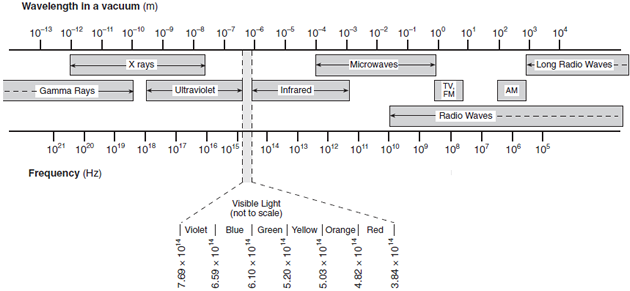# Regents Physics - Electromagnetic Spectrum

## EM Waves

Unlike mechanical waves, electromagnetic (EM) waves do not require a medium in which to travel. They consist of an electric field component and a magnetic field component oriented perpendicular to each other and to the wave velocity, and are caused by vibrating electrical charges. The orientation of the electric field and magnetic field components of an electromagnetic wave can be visualized below, with the red lines representing the electric field and the blue lines representing the magnetic field.

Courtesy Penn State Schuylkill

## EM Wave Speed

The speed of all electromagnetic waves in a vacuum is approximately 3*108 m/s, so important this speed is represented by the letter c. This is, according to our current understanding of the universe, the fastest possible speed anything in the universe can travel.

Because the speed of all electromagnetic waves in a vacuum is c, we can re-write the wave equation as follows:Since c is a constant for all EM waves in a vacuum, the product of frequency and wavelength must be a constant. Therefore, at higher frequencies, EM waves have a shorter wavelength, and at lower frequencies, EM waves have a longer wavelength. If the EM wave travels into a new medium, its speed can be decreased (see index of refraction), and because frequency remains constant, its wavelength would also decrease.

## EM Spectrum

It is the frequency of an EM wave that determines its characteristics. The relationship between frequency and wavelength in a vacuum for various types of EM waves is depicted in the Electromagnetic Spectrum, a diagram which is given in Regents Physics Reference Table:You can use the electromagnetic spectrum to answer questions about EM waves.

## Energy of an EM Wave

The electromagnetic spectrum describes the types of electromagnetic waves observed at the specified frequencies and wavelength. It is also important to note that the energy of an electromagnetic wave is directly related to its frequency, therefore higher frequency (shorter wavelength) EM waves have more energy than lower frequency (longer wavelength) EM waves.An x-ray, therefore, has considerably more energy than an AM radio wave! Using the diagram, more energetic waves are shown on the left of the EM Spectrum diagram, and less energetic waves are shown to the right on the EM Spectrum. We'll explore the energy of EM radiation further in our Modern Physics unit.